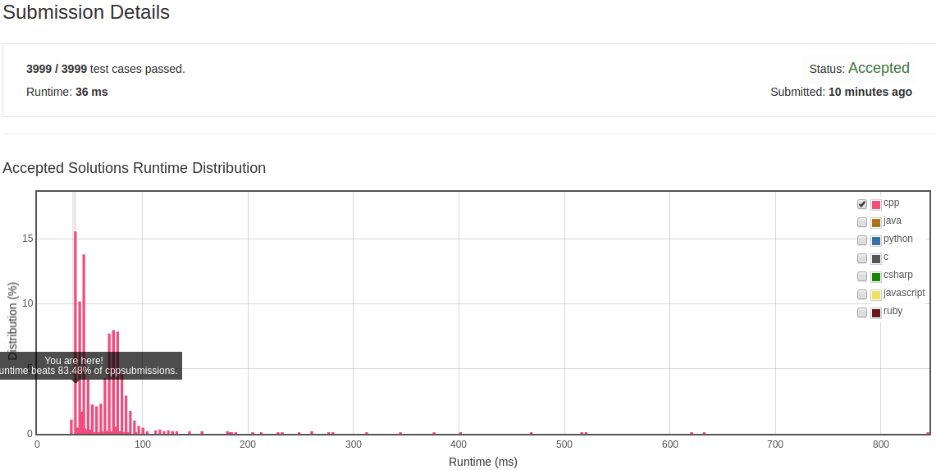# 凌云的博客

## LeetCode 算法题 13. 罗马数字转整数

### 题目

``````字符          数值
I             1
V             5
X             10
L             50
C             100
D             500
M             1000
``````

• I 可以放在 V (5) 和 X (10) 的左边，来表示 4 和 9。
• X 可以放在 L (50) 和 C (100) 的左边，来表示 40 和 90。
• C 可以放在 D (500) 和 M (1000) 的左边，来表示 400 和 900。

``````输入: "III"

``````

``````输入: "IV"

``````

``````输入: "IX"

``````

``````输入: "LVIII"

``````

``````输入: "MCMXCIV"

``````

### 解法

Wikipedia: Roman numerals

``````class Solution {
public:
int romanToInt(string s) {
if (s.empty()) {
return 0;
}

int romans;
romans['I' - 'A'] = 1;
romans['V' - 'A'] = 5;
romans['X' - 'A'] = 10;
romans['L' - 'A'] = 50;
romans['C' - 'A'] = 100;
romans['D' - 'A'] = 500;
romans['M' - 'A'] = 1000;

int result = 0;
int cur = romans[s - 'A'], right = 0;
const int size = s.size();

for (int i = 1; i != size; ++i) {
right = romans[s[i] - 'A'];
if (cur < right) {
result -= cur;
} else {
result += cur;
}

cur = right;
}

result += cur;
return result;
}
};
``````